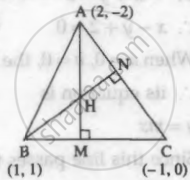Advertisement Remove all ads

# Find the coordinates of the orthocenter of the triangle whose vertices are A(2, −2), B(1, 1), and C(−1, 0). - Mathematics and Statistics

Sum

Find the coordinates of the orthocenter of the triangle whose vertices are A(2, −2), B(1, 1), and C(−1, 0).

Advertisement Remove all ads

#### SolutionLet AM and BN be the altitudes of the ΔABC.

Now, slope of BC = (0 - 1)/(-1 - 1) = 1/2

Altitude AM a perpendicular to side BC.

∴ slope of altitude AM = – 2 and it is passing through A(2, –2).

∴ equation of the altitude AM is y – (– 2) = – 2 (x – 2)

∴ y + 2 = – 2x + 4

∴ 2x + y = 2     ...(1)

Slope of side AC = (0 - (- 2))/(-1 - 2) = -2/3

Altitude BN is perpendicular to side AC.

∴ slope of altitude BN = 3/2 and it is passing through B (1, 1).

∴ equation of the altitude BN is y – 1 = 3/2(x - 1)

∴ 2y – 2 = 3x – 3

∴ 3x – 2y = 1   ...(2)

The orthocentre H is the point of intersection of the altitudes AM and BN. Hence, we solve equations (1) and (2).

Multiply equation (1) by 2, we get,

4x + 2y = 4    ...(3)

Adding (2) and (3), we get,

7x = 5

∴  x = 5/7

∴  from (1), 2(5/2) + y = 2

∴ y = 2 - 10/7

= 4/7

Hence, coordinates of orthocentre H are (5/7, 4/7).

Is there an error in this question or solution?
Advertisement Remove all ads

#### APPEARS IN

Advertisement Remove all ads
Advertisement Remove all ads
Share
Notifications

View all notifications

Forgot password?